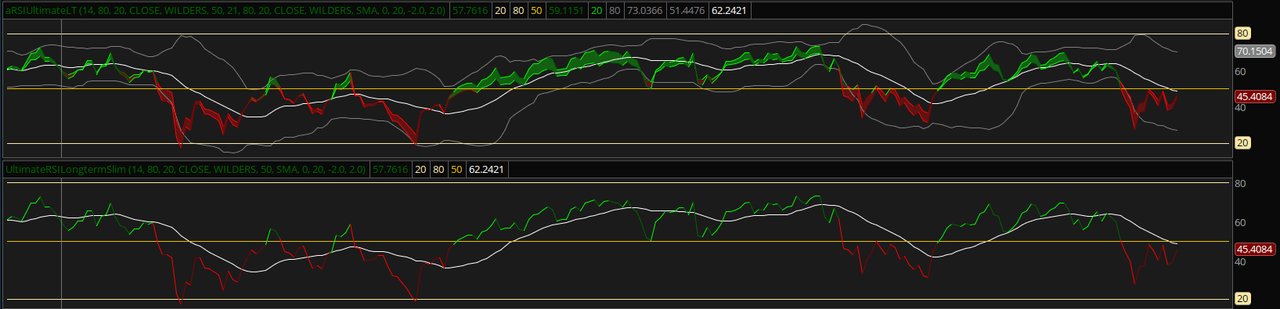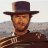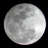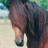# Ultimate RSI Indicator for ThinkorSwim

C

#### Craighaber71

2019 Donor
Warehouse
VIP
That was it...

L

##### New member
Warehouse
Hi, sorry forgot all about this thread. My apologies also for clogging and hijacking your thread - that was not my intention though it is obvious now that I think about it ! Just was excited that a) I finished all those connors scripts which led me to search for other style rsi indicators to begin with And b) was trying to contribute back and swap studies. I will anwer the questions though if you don’t mind and that will be it on this thread.

Hey I really like you rsi Indiator looks great and works great. I like the stripped version best with the short term settings as well even on longer time frames personally. Any way my DTEK studies should work that I I linked. Have the “hide on in intraday” line of code in them all as connor strategies are meant for daily charts. But you can delete the first or second “declare hide in intraday” lines in each study and it will then show up on all charts. I haven’t used his strategies FYI - just read his books last weekend and went ahead and coded the strategies and added labeled to see if I like them . The strategies and PDFs can be found on scribed. Hope that helps and that’s it’s about those studies on this thread. My apologies again.

Thanks!

•Joreha and markos#### markos

##### Well-known member
VIP
@leakywaders You're ok, I recently hijacked another thread myself. It was your first post? Very good! I started a Connors RSI2 thread for you to use.
Feel free to watch it and answer questions there as well. I would have posted more there but my CPU fried.
Start a new thread whenever you have a new study to bring up.Thanks, Markos

T

#### thinky

##### Member
@horserider Noticed you using the long-term version for the 1D, 15m, and 5m charts. Do you use the short-term version with 1m charts?#### horserider

##### Well-known member
VIP
@thinky The long and short term difference is the inputs used. Either can be used on any time frame chart. The long will have higher inputs so will be slower reacting to price changes but as such will be less choppy. So for trading it will signal a bit later and avoid choppiness and keep you in a trade a bit longer. I do not trade 1m. Just experiment and see what works for you. Put both on a chart together and compare. Change inputs if you want. The idea is to set it up the way you like and the way it makes you profits.

••doeman, thinky and markos
T

#### thinky

##### Member
@horserider What is the interpretation of the RSI crossing the SMA line? An early signal of a possible change in trend?#### horserider

##### Well-known member
VIP
@thinky RSI crossing the midline is the signal for a change. I did a chart. This is the long term with higher inputs. Maybe that will show it better than I can write an explanation.So the reversal signal is the RSI crossing the midline. Remember the 50 line is the break from strength to weakness. So you can trade just the signals or use the other information to better inform your decisions.

Now short term. You can see the difference. Possibly just trading the signal is best. Also I stripped out the other info and just showed RSI / midline cross. Might give a clearer example of signals.••MerryDay, rovo, princesly and 4 others
D

#### David45

##### Member
2019 Donor
Hello horesrider,

At the top of this thread you posted your chart which shows the Ultimate RSI:

Short term
Long term
Skeleton of RSI and SMA short term

I was wondering if you had a study for: Skeleton of RSI and SMA long term that you could share? These studies look really great and thank you so much for sharing them!#### horserider

##### Well-known member
VIP
@David45 If you have the one shared and that you quoted above, just edit studies and change the length and then the lengthbb to whatever you wish. The length is for the RSI and lengthbb is for the RSI average. Maybe you want to try 14 for both. Then experiment till you get something that fits your trading. I think you said long term so go higher and see how it fits.

Ok did a quick look, try 30 and 34. Remember the 50 line is also important for RSI.

•David45
T

#### thinky

##### Member
@David45 This is the skeleton version of the long-term version: http://tos.mx/ttVLIj•David45#### mc01439

##### Well-known member
2019 Donor
VIP
A double RSI indicator with standard deviation bands of the RSI. The very important RSI 50 line and coloring of the RSI lines for strength and weakness with a could to show when the two RSI lines separate. This would indicate a stronger move.

The Midline of the RSI bands works the same as the Market Mover with crossover of the RSI and Midline giving the signals.

The bands and 80 / 20 lines will show when RSI is very strong in one direction or the other. It can be traded the same as BBs by trading from top to bottom band and vice versa, that would be for the most adventurous trader.

The cross of the RSI and Midline would be the next step down in risk for a trade, while that same cross along with a cross of the 50 line would be the conservative trade entry or exit.

The RSI is not an oversold/overbought indicator, it is a strength indicator. So when RSI is high it is telling you the trend is strong, low shows trend is weak. So even when high and falling if it does not break the 50 line the up trend is most likely to continue. So always keep in mind the importance of the 50 line.

I will put up two versions one for scalping types which will match Market Mover signals (without scaling problems and zooming) and one for swing trading types.

Any feedback or questions are welcome.

Short term
Long term
Skeleton of RSI and SMA short termShort term
http://tos.mx/N6WBa1

Long term
http://tos.mx/lpvzpv
@horserider - Nice way to use RSI. Your method gives a lot more insight into market movement possibilities. The time tested indicators just seem to do the job better. Thanks for sharing.

D

#### David45

##### Member
2019 Donor
@David45 This is the skeleton version of the long-term version: http://tos.mx/ttVLIjThank you for sharing thinky!

D

#### David45

##### Member
2019 Donor
@David45 If you have the one shared and that you quoted above, just edit studies and change the length and then the lengthbb to whatever you wish. The length is for the RSI and lengthbb is for the RSI average. Maybe you want to try 14 for both. Then experiment till you get something that fits your trading. I think you said long term so go higher and see how it fits.

Ok did a quick look, try 30 and 34. Remember the 50 line is also important for RSI.
Thank you so much horserider! I will try these settings. I have some follow up questions if you would kindly reply:

What causes the lines turn from green to red and red to green? How about light green to dark green and light red to dark red?

If using this indicator for longer term trades, could you simply go long when the lines are light green or dark green and go short when the lines are light red or dark red? Or is the idea to go long when the lines are light green or dark green AND above the zero line or go short when the lines are light red or dark red AND below the zero line? It seems that using the color of the lines alone gets you in and out of the trend earlier, but I guess the zero line crossing is further confirmation even if it gets you in later?

Are there any other nuances I'm missing especially in regards to knowing when a signal is false so that you don't get whipsawed?

Thank you again for sharing this fantastic indicator!#### horserider

##### Well-known member
VIP
There is no zero line. Maybe you mean the midline (white line). The white line is the signal line. The important lines are the midline and 50 line. You can choose when to take a trade depending on your risk tolerance. Take a look at the chart posted earlier with annotations of possible ways to trade the indicator. ( POST # 27 ) Of course the longer time frame you use and the longer lengths on the indicator should help with avoiding quick reversals. Wish I knew a way to avoid them but do not.

The RSI lines change colors when they cross the 50 line.
If RSI is above 50 line and going up it is bright green. Over 50 line and going down it is dark green.
If RSI is below 50 line and going down it is bright red. Below 50 Line and going up it is dark red.

Say you use 14, 21 for the RSI lengths, the cloud will turn green when the 14 is above the 21 and the cloud will turn red when the 14 is below the 21.
When the cloud is very narrow or no cloud there is consolidation. Same with the bands, narrowing of bands is consolidation. Expansion of cloud or bands is more volatile.

••rovo, markos, thinky and 1 other person
D

#### David45

##### Member
2019 Donor
There is no zero line. Maybe you mean the midline (white line). The white line is the signal line. The important lines are the midline and 50 line. You can choose when to take a trade depending on your risk tolerance. Take a look at the chart posted earlier with annotations of possible ways to trade the indicator. ( POST # 27 ) Of course the longer time frame you use and the longer lengths on the indicator should help with avoiding quick reversals. Wish I knew a way to avoid them but do not.

The RSI lines change colors when they cross the 50 line.
If RSI is above 50 line and going up it is bright green. Over 50 line and going down it is dark green.
If RSI is below 50 line and going down it is bright red. Below 50 Line and going up it is dark red.

Say you use 14, 21 for the RSI lengths, the cloud will turn green when the 14 is above the 21 and the cloud will turn red when the 14 is below the 21.
When the cloud is very narrow or no cloud there is consolidation. Same with the bands, narrowing of bands is consolidation. Expansion of cloud or bands is more volatile.

Thank you so much horserider! You answered all of my questions exactly. Now it makes sense. Very much appreciated!

D

#### Darrenkohcc

##### New member
thank you
Hi Tanp82, horserider,

I loaded the candlestick label script, don't see that it is working, able to advise and assist?#### Birdie

##### New member
2019 Donor
Warehouse
Just posting the actual code here for import
Short term ultimate RSI:

Code:
``````# RSI Bands and Double RSI SMA Cross Short Term Trades
#Created by Horserider 7/20/2019

declare lower;
#RSI
input length = 5;
input over_Bought = 80;
input over_Sold = 20;
input price = close;
input averageType = AverageType.WILDERS;
input line = 50;

def NetChgAvg = MovingAverage(averageType, price - price, length);
def TotChgAvg = MovingAverage(averageType, AbsValue(price - price), length);
def ChgRatio = if TotChgAvg != 0 then NetChgAvg / TotChgAvg else 0;

plot RSI = 50 * (ChgRatio + 1);
plot OverSold = over_Sold;
plot OverBought = over_Bought;
plot lline = 50;

#                ==== THE FIVE ====
RSI.DefineColor("Positive and Up", Color.GREEN);
RSI.DefineColor("Positive and Down", Color.DARK_GREEN);
RSI.DefineColor("Negative and Down", Color.RED);
RSI.DefineColor("Negative and Up", Color.DARK_RED);
RSI.AssignValueColor(if RSI >= 50 then if RSI > RSI then RSI.Color("Positive and Up") else RSI.Color("Positive and Down") else if RSI < RSI then RSI.Color("Negative and Down") else RSI.Color("Negative and Up"));

OverSold.SetDefaultColor(GetColor(8));
OverBought.SetDefaultColor(GetColor(8));

# RSI 2
input length2 = 8;
input over_Bought2 = 80;
input over_Sold2 = 20;
input price2 = close;
input averageType2 = AverageType.WILDERS;

def NetChgAvg2 = MovingAverage(averageType2, price2 - price2, length2);
def TotChgAvg2 = MovingAverage(averageType2, AbsValue(price2 - price2), length2);
def ChgRatio2 = if TotChgAvg2 != 0 then NetChgAvg2 / TotChgAvg2 else 0;

plot RSI2 = 50 * (ChgRatio2 + 1);
plot OverSold2 = over_Sold;
plot OverBought2 = over_Bought;

RSI2.DefineColor("Positive and Up", Color.GREEN);
RSI2.DefineColor("Positive and Down", Color.DARK_GREEN);
RSI2.DefineColor("Negative and Down", Color.RED);
RSI2.DefineColor("Negative and Up", Color.DARK_RED);
RSI2.AssignValueColor(if RSI2 >= 50 then if RSI2 > RSI2 then RSI2.Color("Positive and Up") else RSI2.Color("Positive and Down") else if RSI2 < RSI2 then RSI2.Color("Negative and Down") else RSI2.Color("Negative and Up"));

#plot 5;

input AverageTypeBB = {default SMA, EMA, HMA};
input displaceBB = 0;
input lengthBB = 5;
input Num_Dev_Dn = -2.0;
input Num_Dev_up = 2.0;

plot upperBand;
plot lowerBand;
plot midline ;

switch (AverageTypeBB) {
case SMA:
upperBand = reference BollingerBands(RSI, displaceBB, lengthBB, Num_Dev_Dn, Num_Dev_up).UpperBand;
lowerBand = reference BollingerBands(RSI, displaceBB, lengthBB, Num_Dev_Dn, Num_Dev_up).LowerBand;
midline   = reference BollingerBands(RSI, displaceBB, lengthBB, Num_Dev_Dn, Num_Dev_up).Midline;
case EMA:
upperBand = reference BollingerBands(RSI, displaceBB, lengthBB, Num_Dev_Dn, Num_Dev_up, averageType = AverageType.EXPONENTIAL).UpperBand;
lowerBand = reference BollingerBands(RSI, displaceBB, lengthBB, Num_Dev_Dn, Num_Dev_up, averageType = AverageType.EXPONENTIAL).LowerBand;
midline   = reference BollingerBands(RSI, displaceBB, lengthBB, Num_Dev_Dn, Num_Dev_up, averageType = AverageType.EXPONENTIAL).Midline;
case HMA:
upperBand = reference BollingerBands(RSI, displaceBB, lengthBB, Num_Dev_Dn, Num_Dev_up, averageType = AverageType.EXPONENTIAL).UpperBand;
lowerBand = reference BollingerBands(RSI, displaceBB, lengthBB, Num_Dev_Dn, Num_Dev_up, averageType = AverageType.EXPONENTIAL).LowerBand;
midline   = reference BollingerBands(RSI, displaceBB, lengthBB, Num_Dev_Dn, Num_Dev_up, averageType = AverageType.EXPONENTIAL).Midline;
}

upperBand.SetDefaultColor(Color.GRAY);

lowerBand.SetDefaultColor(Color.GRAY);

midline.SetDefaultColor(Color.WHITE);``````

•BenTen#### Birdie

##### New member
2019 Donor
Warehouse
Long Term Ultimate RSI

Code:
``````# RSI Bands and Double RSI SMA Cross Long Term Trades
#Created by Horserider 7/20/2019

declare lower;
#RSI
input length = 14;
input over_Bought = 80;
input over_Sold = 20;
input price = close;
input averageType = AverageType.WILDERS;
input line = 50;

def NetChgAvg = MovingAverage(averageType, price - price, length);
def TotChgAvg = MovingAverage(averageType, AbsValue(price - price), length);
def ChgRatio = if TotChgAvg != 0 then NetChgAvg / TotChgAvg else 0;

plot RSI = 50 * (ChgRatio + 1);
plot OverSold = over_Sold;
plot OverBought = over_Bought;
plot lline = 50;

#                ==== THE FIVE ====
RSI.DefineColor("Positive and Up", Color.GREEN);
RSI.DefineColor("Positive and Down", Color.DARK_GREEN);
RSI.DefineColor("Negative and Down", Color.RED);
RSI.DefineColor("Negative and Up", Color.DARK_RED);
RSI.AssignValueColor(if RSI >= 50 then if RSI > RSI then RSI.Color("Positive and Up") else RSI.Color("Positive and Down") else if RSI < RSI then RSI.Color("Negative and Down") else RSI.Color("Negative and Up"));

OverSold.SetDefaultColor(GetColor(8));
OverBought.SetDefaultColor(GetColor(8));

# RSI 2
input length2 = 21;
input over_Bought2 = 80;
input over_Sold2 = 20;
input price2 = close;
input averageType2 = AverageType.WILDERS;

def NetChgAvg2 = MovingAverage(averageType2, price2 - price2, length2);
def TotChgAvg2 = MovingAverage(averageType2, AbsValue(price2 - price2), length2);
def ChgRatio2 = if TotChgAvg2 != 0 then NetChgAvg2 / TotChgAvg2 else 0;

plot RSI2 = 50 * (ChgRatio2 + 1);
plot OverSold2 = over_Sold;
plot OverBought2 = over_Bought;

RSI2.DefineColor("Positive and Up", Color.GREEN);
RSI2.DefineColor("Positive and Down", Color.DARK_GREEN);
RSI2.DefineColor("Negative and Down", Color.RED);
RSI2.DefineColor("Negative and Up", Color.DARK_RED);
RSI2.AssignValueColor(if RSI2 >= 50 then if RSI2 > RSI2 then RSI2.Color("Positive and Up") else RSI2.Color("Positive and Down") else if RSI2 < RSI2 then RSI2.Color("Negative and Down") else RSI2.Color("Negative and Up"));

#plot 5;

input AverageTypeBB = {default SMA, EMA, HMA};
input displaceBB = 0;
input lengthBB = 20;
input Num_Dev_Dn = -2.0;
input Num_Dev_up = 2.0;

plot upperBand;
plot lowerBand;
plot midline ;

switch (AverageTypeBB) {
case SMA:
upperBand = reference BollingerBands(RSI, displaceBB, lengthBB, Num_Dev_Dn, Num_Dev_up).UpperBand;
lowerBand = reference BollingerBands(RSI, displaceBB, lengthBB, Num_Dev_Dn, Num_Dev_up).LowerBand;
midline   = reference BollingerBands(RSI, displaceBB, lengthBB, Num_Dev_Dn, Num_Dev_up).Midline;
case EMA:
upperBand = reference BollingerBands(RSI, displaceBB, lengthBB, Num_Dev_Dn, Num_Dev_up, averageType = AverageType.EXPONENTIAL).UpperBand;
lowerBand = reference BollingerBands(RSI, displaceBB, lengthBB, Num_Dev_Dn, Num_Dev_up, averageType = AverageType.EXPONENTIAL).LowerBand;
midline   = reference BollingerBands(RSI, displaceBB, lengthBB, Num_Dev_Dn, Num_Dev_up, averageType = AverageType.EXPONENTIAL).Midline;
case HMA:
upperBand = reference BollingerBands(RSI, displaceBB, lengthBB, Num_Dev_Dn, Num_Dev_up, averageType = AverageType.EXPONENTIAL).UpperBand;
lowerBand = reference BollingerBands(RSI, displaceBB, lengthBB, Num_Dev_Dn, Num_Dev_up, averageType = AverageType.EXPONENTIAL).LowerBand;
midline   = reference BollingerBands(RSI, displaceBB, lengthBB, Num_Dev_Dn, Num_Dev_up, averageType = AverageType.EXPONENTIAL).Midline;
}

upperBand.SetDefaultColor(Color.GRAY);

midline.setDefaultColor(Color.WHITE);

lowerBand.SetDefaultColor(Color.GRAY);``````

•BenTen
D

#### doeman

##### New member
2019 Donor
Thank You. Looks great

T

#### Tostechnical

##### Member
So I made a small edit to the Ultimate RSI. Edited the the bands so that they would turn magenta when they expand. I found that a 5-14 setting for the RSI with the SMA set to 20 seems the be the sweet spot for daily chart. I use it with the MOBO

Code:
``````declare lower;
#RSI
input length = 5;
input over_Bought = 80;
input over_Sold = 20;
input price = close;
input averageType = AverageType.WILDERS;
input line = 50;

def NetChgAvg = MovingAverage(averageType, price - price, length);
def TotChgAvg = MovingAverage(averageType, AbsValue(price - price), length);
def ChgRatio = if TotChgAvg != 0 then NetChgAvg / TotChgAvg else 0;

plot RSI = 50 * (ChgRatio + 1);
plot OverSold = over_Sold;
plot OverBought = over_Bought;
plot lline = 50;

#                ==== THE FIVE ====
RSI.DefineColor("Positive and Up", Color.GREEN);
RSI.DefineColor("Positive and Down", Color.DARK_GREEN);
RSI.DefineColor("Negative and Down", Color.RED);
RSI.DefineColor("Negative and Up", Color.DARK_RED);
RSI.AssignValueColor(if RSI >= 50 then if RSI > RSI then RSI.Color("Positive and Up") else RSI.Color("Positive and Down") else if RSI < RSI then RSI.Color("Negative and Down") else RSI.Color("Negative and Up"));

OverSold.SetDefaultColor(GetColor(8));
OverBought.SetDefaultColor(GetColor(8));

# RSI 2
input length2 = 14;
input over_Bought2 = 80;
input over_Sold2 = 20;
input price2 = close;
input averageType2 = AverageType.WILDERS;

def NetChgAvg2 = MovingAverage(averageType2, price2 - price2, length2);
def TotChgAvg2 = MovingAverage(averageType2, AbsValue(price2 - price2), length2);
def ChgRatio2 = if TotChgAvg2 != 0 then NetChgAvg2 / TotChgAvg2 else 0;

plot RSI2 = 50 * (ChgRatio2 + 1);
plot OverSold2 = over_Sold;
plot OverBought2 = over_Bought;

RSI2.DefineColor("Positive and Up", Color.GREEN);
RSI2.DefineColor("Positive and Down", Color.DARK_GREEN);
RSI2.DefineColor("Negative and Down", Color.RED);
RSI2.DefineColor("Negative and Up", Color.DARK_RED);
RSI2.AssignValueColor(if RSI2 >= 50 then if RSI2 > RSI2 then RSI2.Color("Positive and Up") else RSI2.Color("Positive and Down") else if RSI2 < RSI2 then RSI2.Color("Negative and Down") else RSI2.Color("Negative and Up"));

#plot 5;

input AverageTypeBB = {default SMA, EMA, HMA};
input displaceBB = 0;
input lengthBB = 20;
input Num_Dev_Dn = -2.0;
input Num_Dev_up = 2.0;

plot upperBand;
plot lowerBand;
plot midline ;

switch (AverageTypeBB) {
case SMA:
upperBand = reference BollingerBands(RSI, displaceBB, lengthBB, Num_Dev_Dn, Num_Dev_up).UpperBand;
lowerBand = reference BollingerBands(RSI, displaceBB, lengthBB, Num_Dev_Dn, Num_Dev_up).LowerBand;
midline   = reference BollingerBands(RSI, displaceBB, lengthBB, Num_Dev_Dn, Num_Dev_up).Midline;
case EMA:
upperBand = reference BollingerBands(RSI, displaceBB, lengthBB, Num_Dev_Dn, Num_Dev_up, averageType = AverageType.EXPONENTIAL).UpperBand;
lowerBand = reference BollingerBands(RSI, displaceBB, lengthBB, Num_Dev_Dn, Num_Dev_up, averageType = AverageType.EXPONENTIAL).LowerBand;
midline   = reference BollingerBands(RSI, displaceBB, lengthBB, Num_Dev_Dn, Num_Dev_up, averageType = AverageType.EXPONENTIAL).Midline;
case HMA:
upperBand = reference BollingerBands(RSI, displaceBB, lengthBB, Num_Dev_Dn, Num_Dev_up, averageType = AverageType.EXPONENTIAL).UpperBand;
lowerBand = reference BollingerBands(RSI, displaceBB, lengthBB, Num_Dev_Dn, Num_Dev_up, averageType = AverageType.EXPONENTIAL).LowerBand;
midline   = reference BollingerBands(RSI, displaceBB, lengthBB, Num_Dev_Dn, Num_Dev_up, averageType = AverageType.EXPONENTIAL).Midline;
}

UpperBand.assignValueColor(if absValue(UpperBand-lowerBand)> absValue(UpperBand-lowerBand) then color.magenta else color.gray);
midline.setDefaultColor(Color.WHITE);
lowerband.assignValueColor(if absValue(UpperBand-lowerBand)> absValue(UpperBand-lowerBand) then color.magenta else color.gray);``````

•BenTenIndicators 631Indicators 32Indicators 59